# RF-CONCRETE Surfaces – Online Manual Version 5

Online manuals, introductory examples, tutorials, and other documentation.

# 2.4.1 Design Internal Forces

### Design Internal Forces

The most important formulas for determining the design axial forces from the principal axial forces are presented in Equation 2.5 through Equation 2.7 in chapter 2.3. According to Baumann , these formulas can also be used for moments, because they are nothing more than a force couple with the same absolute value, situated at a certain distance from each other, and with a diametrical direction.

Among other things, plates differ from walls in that the actions result in stresses with different algebraic signs on the opposing sides of the plate. It would therefore make sense to provide plates with reinforcement meshes with different directions for both sides of the plates. Since the principal moments m1 and m2 are determined in the centroidal plane of the surface, they must be distributed to the plate sides in order to be able to determine the design moments for the reinforcement of the respective plate side.

We look at a plate element with its loading. The surface's local coordinate system is located in the centroidal plane of the plate.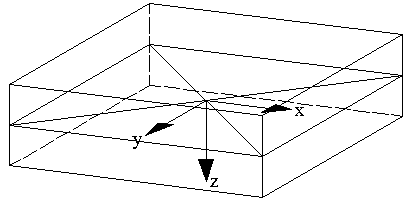Figure 2.24 Plate element with local surface coordinate system in centroidal plane of the plate

In RFEM, the surface's bottom side always lies in the direction of the positive local surface axis z, the top side accordingly in the direction of the negative local z-axis.

Top and bottom side

The surface axes can be switched on in the Display navigator by selecting Model Surfaces Surface Axis Systems x,y,z or in the shortcut menu of surfaces (see Figure 3.28).

In RFEM, the principal internal forces m1 and m2 are determined for the centroidal plane of the plate.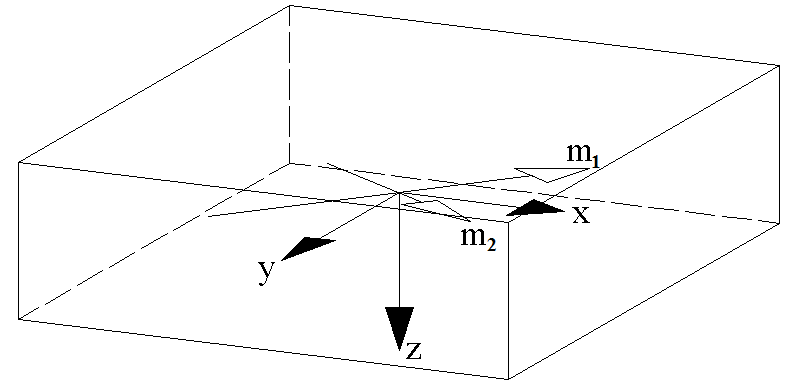Figure 2.25 Principal moments m1 and m2 in the centroidal plane of the plate

The principal moments are displayed as simple arrows. They are oriented like the reinforcement that would be required to resist them. To obtain design moments for the reinforcement mesh at the bottom surface of the plate from these principal moments, the principal moments are shifted to the plate's bottom surface without alteration. For the design, they are described with Roman indexes as mI and mII.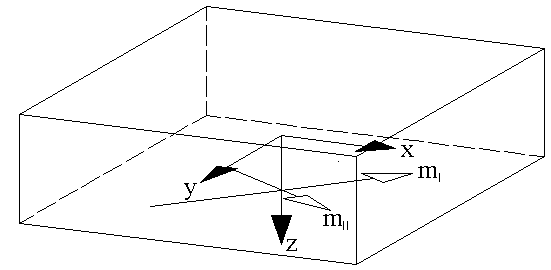Figure 2.26 Principal moments shifted to the bottom side of the plate

To obtain the principal moments for determining the design moments for the reinforcement mesh at the top side of the plate, the principal moments are shifted to the plate's top surface. At the same time, their direction is rotated by 180°.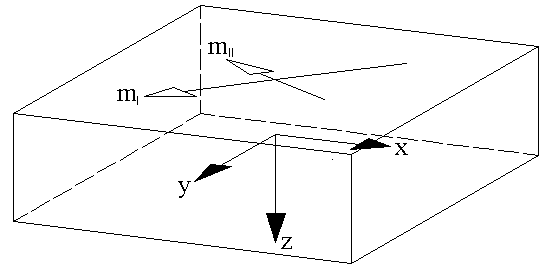Figure 2.27 Principal moments shifted to the top side of the plate

Since the principal moment is usually referred to as m1, which is larger considering the algebraic sign (see Figure 2.27), the denotations of the principal moments at the top side of the plate still have to be reversed.

Thus, the principal moments for determining the design moments at both plate sides are represented as follows: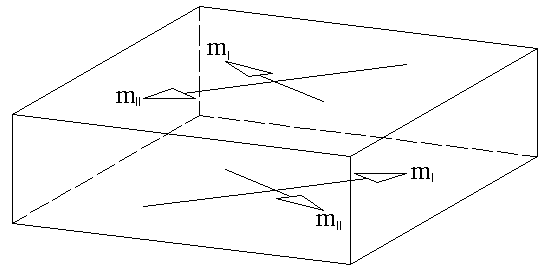Figure 2.28 Final principal moments at bottom and top side of the plate

If the principal moments for both plate sides are known, the design moments can be determined. To that end, the first step is to determine the differential angle of the reinforcement directions to the direction of the principal moment at each plate side.

The smallest differential angle specifies the positive (clockwise) direction. All other angles are determined in this positive direction and then sorted by size. In RF-CONCRETE Surfaces, they are labelled αm,+z, βm,+z, and γm,+z as shown in the following example. The index +z indicates the bottom surface.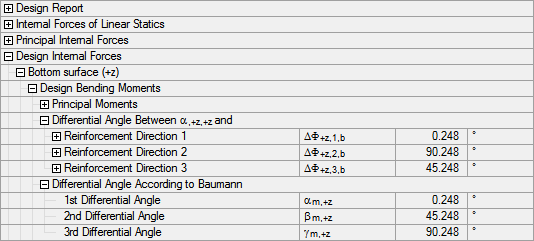Figure 2.29 Differential angle according to  for bottom surface of plate (here for 3 reinforcement directions)

Then, Equations 2.5 through 2.7 are used according to Baumann  in order to determine the design moments:

In RF-CONCRETE Surfaces, the design moments mα,+z, mβ,+z, and mγ,+z for the bottom surface of the plate are output as follows: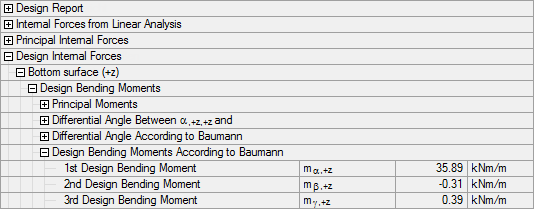Figure 2.30 Design moments according to  for the bottom surface of the plate

In this example, one of the design moments is less than zero. The program now searches for a reinforcement mesh consisting of two reinforcement layers that is stiffened by a concrete compression strut.

The first assumed reinforcement mesh consists of the two reinforcement sets in the directions αm and βm. The direction γ of the stiffening concrete compression strut (the stiffening moment that produces compression at this side of the plate) is assumed to be exactly between these two reinforcement directions.

With the adapted Equations 2.5 through 2.7, the program once more determines the design moments in the selected reinforcement directions of the mesh and the moment that stiffens them. In the example, the result for the plate's bottom side is the following.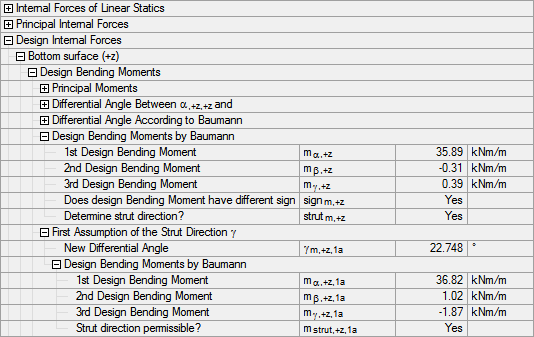Figure 2.31 First assumption for the direction γ of the concrete compression strut

The assumption of the reinforcement mesh results in a viable solution, because the direction of the compression strut is valid.

The analysis of additional compression strut directions must show whether it is the energetic minimum with the least required reinforcement. These analyses are carried out analogously.

Once all sensible possibilities for a reinforcement mesh consisting of two reinforcement directions and a stiffening concrete compression strut are analyzed, the sums of the absolute design moments are shown. For the example above, the overview looks as follows.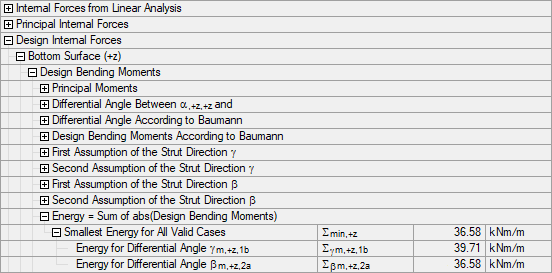Figure 2.32 Sum of the absolute design moments

The Smallest Energy for all Valid Cases ∑min,+z is given as the minimum absolute sum of the determined design moments. In the example, the reinforcement mesh from the reinforcement layouts for the differential angle βm,+z,2a yields the most favorable solution for the bottom side of the plate.

The design details also show the direction of the governing compression strut. This direction is related to the definition of the differential angles according to Baumann. Hence, the program also gives the direction φstrut in relation to the reinforcement direction. In the example, the following compression strut angle is determined for the plate's bottom side: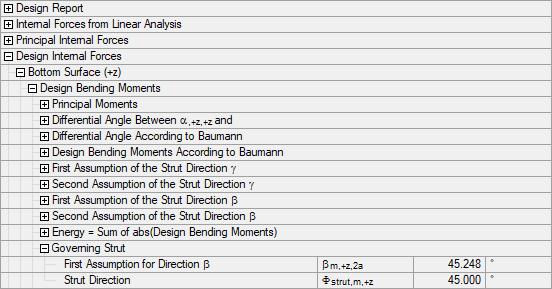Figure 2.33 Governing compression strut

For an optimized direction of the design moment that stiffens the reinforcement mesh (see Figure 3.47), the design moments according to Baumann are obtained for the example above. These design moments are applied to the defined reinforcement directions as shown in the following figure.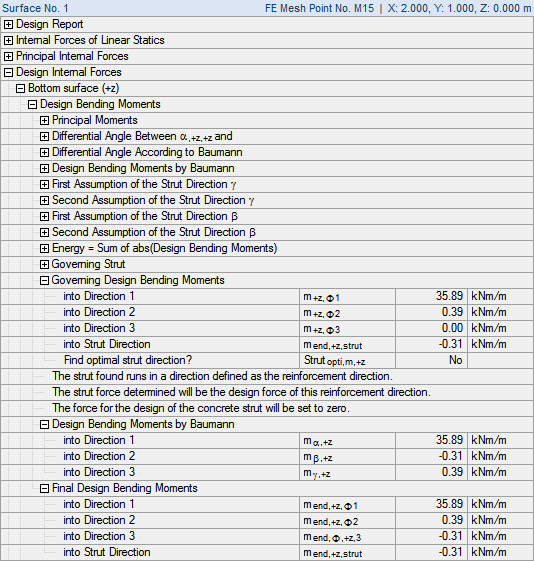Figure 2.34 Final design moments for bottom side of plate
Literatur
  Deutscher Ausschuss für Stahlbeton, Heft 217: Tragwirkung orthogonaler Bewehrungsnetze beliebiger Richtung in Flächentragwerken aus Stahlbeton (von Theodor Baumann). Verlag Ernst & Sohn, Berlin, 1972.